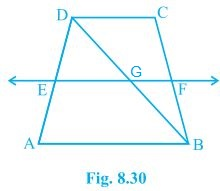# ABCD is a trapezium in which AB || DC, BD is a diagonal and E is the mid-point of AD. A line is drawn through E parallel to AB intersecting BC at F (see Fig.) . Show that F is the mid-point of BC.Given that

ABCD is a trapezium in which AB || DC, BD is a diagonal and E is the mid-point of AD.

To prove

F is the mid-point of BC.

Proof

BD intersected EF at G.

E is the mid point of AD and also EG || AB.

Thus, G is the mid point of BD (Converse of mid point theorem)

Now,

From ΔBDC,

G is the mid point of BD and also GF || AB || DC.

Thus, F is the mid point of BC (Converse of mid point theorem)